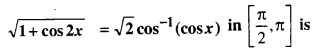# Bihar Board 12th Maths Objective Answers Chapter 2 Inverse Trigonometric Functions

Bihar Board 12th Maths Objective Questions and Answers

## Bihar Board 12th Maths Objective Answers Chapter 2 Inverse Trigonometric Functions

Question 1.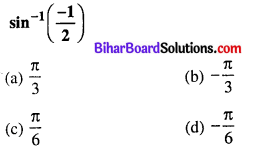(d) $$-\frac{\pi}{6}$$

Question 2.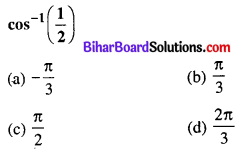(b) $$\frac{\pi}{3}$$

Question 3.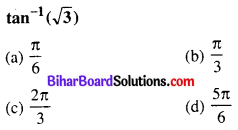(b) $$\frac{\pi}{3}$$

Question 4.(a) $$\frac{\pi}{4}$$

Question 5.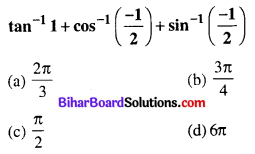(b) $$\frac{3 \pi}{4}$$

Question 6.(d) $$\frac{2 \pi}{3}$$

Question 7.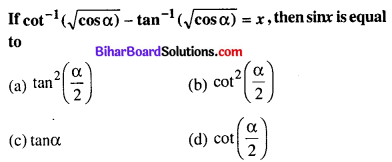(a) $$\tan ^{2}\left(\frac{\alpha}{2}\right)$$

Question 8.(b) $$\frac{6}{17}$$

Question 9.
If tan-1 (x – 1) + tan-1 x + tan-1 (x + 1) = tan-1 3x, then the values of x are
(a) $$\pm \frac{1}{2}$$
(b) 0, $$\frac{1}{2}$$
(c) 0, $$-\frac{1}{2}$$
(d) 0, $$\pm \frac{1}{2}$$
(d) 0, $$\pm \frac{1}{2}$$

Question 10.
If 6sin-1 (x2 – 6x + 8.5) = π, then x is equal to
(a) 1
(b) 2
(c) 3
(d) 8
(b) 2

Question 11.
3 tan-1 a is equal to(d) $$\tan ^{-1}\left(\frac{3 a-a^{3}}{1-3 a^{2}}\right)$$

Question 12.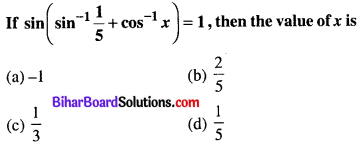(d) $$\frac{1}{5}$$

Question 13.
The equation 2cos-1 x + sin-1 x = $$\frac{11 \pi}{6}$$ has
(a) no solution
(b) only one solution
(c) two solutions
(d) three solutions
(a) no solution

Question 14.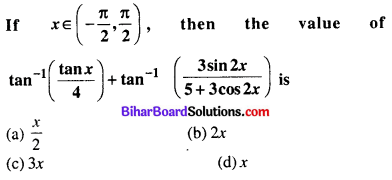(d) x

Question 15.
If tan-1 2x + tan-1 3x = $$\frac{\pi}{4}$$, then x is
(a) $$\frac{1}{6}$$
(b) 1
(c) ($$\frac{1}{6}$$, -1)
(d) none of these
(a) $$\frac{1}{6}$$

Question 16.(c) $$\sqrt{\frac{x^{2}+1}{x^{2}+2}}$$

Question 17.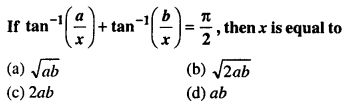(a) $$\sqrt{a b}$$

Question 18.
If tan-1 x – tan-1 y = tan-1 A, then A is equal to
(a) x – y
(b) x + y
(c) $$\frac{x-y}{1+x y}$$
(d) $$\frac{x+y}{1-x y}$$
(c) $$\frac{x-y}{1+x y}$$

Question 19.(c) $$\pm \sqrt{\frac{5}{2}}$$

Question 20.
The value of cot-1 9 + cosec-1($$\frac{\sqrt{41}}{4}$$) is given by
(a) 0
(b) $$\frac{\pi}{4}$$
(c) tan-1 2
(d) $$\frac{\pi}{2}$$
(b) $$\frac{\pi}{4}$$

Question 21.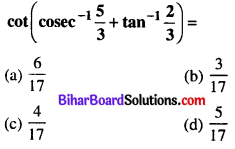(a) $$\frac{6}{17}$$

Question 22.(d) none of these

Question 23.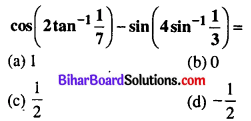(b) 0

Question 24.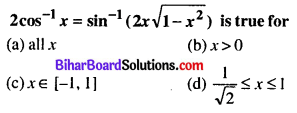(d) $$\frac{1}{\sqrt{2}} \leq x \leq 1$$

Question 25.
cos-1[cos(2cot-1(√2 – 1))] = ______
(a) √2 – 1
(b) 1 + √2
(c) $$\frac{\pi}{4}$$
(d) $$\frac{3 \pi}{4}$$
(d) $$\frac{3 \pi}{4}$$

Question 26.
The range of sin-1 x + cos-1 x + tan-1 x is
(a) [0, π]
(b) $$\left[\frac{\pi}{4}, \frac{3 \pi}{4}\right]$$
(c) (0, π)
(d) $$\left[0, \frac{\pi}{2}\right]$$
(b) $$\left[\frac{\pi}{4}, \frac{3 \pi}{4}\right]$$

Question 27.(c) $$\frac{\pi}{4}$$

Question 28.
Find the value of sec2 (tan-1 2) + cosec2 (cot-1 3)
(a) 12
(b) 5
(c) 15
(d) 9
(c) 15

Question 29.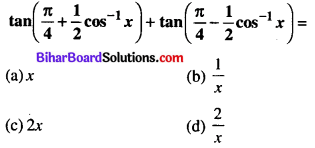(d) $$\frac{2}{x}$$

Question 30.
The equation sin-1 x – cos-1 x = cos-1($$\frac{\sqrt{3}}{2}$$) has
(a) unique solution
(b) no solution
(c) infinitely many solution
(d) none of these
(a) unique solution

Question 31.
Solve for x : {xcos(cot-1 x) + sin(cot-1 x)}2 = $$\frac{51}{50}$$
(a) $$\frac{1}{\sqrt{2}}$$
(b) $$\frac{1}{5 \sqrt{2}}$$
(c) 2√2
(d) 5√2
(b) $$\frac{1}{5 \sqrt{2}}$$

Question 32.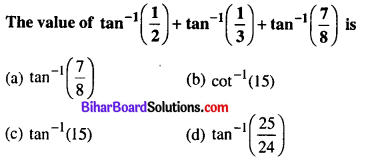(c) tan-1(15)

Question 33.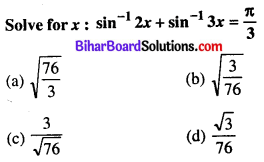(b) $$\sqrt{\frac{3}{76}}$$

Question 34.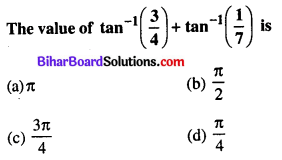(d) $$\frac{\pi}{4}$$

Question 35.
If sin-1(x2 – 7x + 12) = nπ, ∀ n ∈ I, then x =
(a) -2
(b) 4
(c) -3
(d) 5
(b) 4

Question 36.
If cos-1 x + sin-1 x = π, then the value of x is
(a) $$\frac{3}{2}$$
(b) $$\frac{1}{\sqrt{2}}$$
(c) $$\frac{\sqrt{3}}{2}$$
(d) $$\frac{2}{\sqrt{3}}$$
(c) $$\frac{\sqrt{3}}{2}$$

Question 37.
If sin-1 x – cos-1 x = $$\frac{\pi}{6}$$, then x =
(a) $$\frac{1}{2}$$
(b) $$\frac{\sqrt{3}}{2}$$
(c) $$-\frac{1}{2}$$
(d) $$-\frac{\sqrt{3}}{2}$$
(b) $$\frac{\sqrt{3}}{2}$$

Question 38.
If tan-1 (cot θ) = 2θ, then θ is equal to
(a) $$\frac{\pi}{3}$$
(b) $$\frac{\pi}{4}$$
(c) $$\frac{\pi}{6}$$
(d) None of these
(c) $$\frac{\pi}{6}$$

Question 39.
cot($$\frac{\pi}{4}$$ – 2cot-1 3) =
(a) 7
(b) 6
(c) 5
(d) None of these
(a) 7

Question 40.
If tan-1 3 + tan-1 x = tan-1 8, then x =
(a) 5
(b) $$\frac{1}{5}$$
(c) $$\frac{5}{14}$$
(d) $$\frac{14}{5}$$
(b) $$\frac{1}{5}$$

Question 41.(d) $$-\frac{24}{25}$$

Question 42.
sin-1 (1 – x) – 2sin-1 x = $$\frac{\pi}{2}$$
(a) 0
(b) 1/2
(c) 0, 1/2
(d) -1/2
(a) 0

Question 43.
2tan-1(cos x) = tan-1(2cosec x)
(a) 0
(b) π/3
(c) π/4
(d) π/2
(c) π/4

Question 44.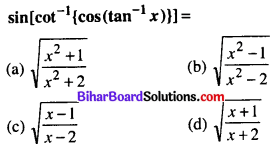(a) $$\sqrt{\frac{x^{2}+1}{x^{2}+2}}$$

Question 45.(a) $$\frac{3 \pi}{5}$$

Question 46.
The domain of the function defind by f(x) = $$\sin ^{-1} \sqrt{x-1}$$ is
(a) [1, 2]
(b) [-1, 1]
(c) [0, 1]
(d) none of these
(a) [1, 2]

Question 47.
The value of sin (2tan-1 (0.75)) is equal to
(a) 0.75
(b) 1.5
(c) 0.96
(d) sin 1.5
(c) 0.96

Question 48.
The value of expression 2 sec-1 2 + sin-1($$\frac{1}{2}$$)
(a) $$\frac{\pi}{6}$$
(b) $$\frac{5 \pi}{6}$$
(c) $$\frac{7 \pi}{6}$$
(d) 1
(b) $$\frac{5 \pi}{6}$$

Question 49.(c) $$\frac{24}{25}$$

Question 50.
The value of the expression $$\tan \left(\frac{1}{2} \cos ^{-1} \frac{2}{\sqrt{3}}\right)$$
(a) 2 + √5
(b) √5 – 2
(c) $$\frac{\sqrt{5}+2}{2}$$
(d) 5 + √2
(b) √5 – 2

Question 51.